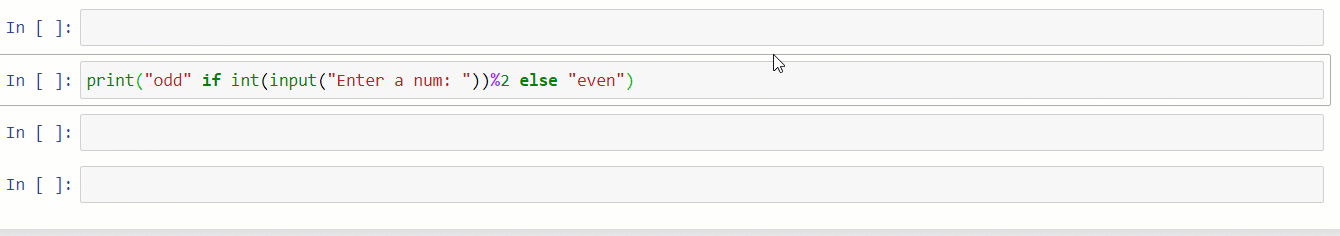Development# 10 Python Tips and Tricks That Will Wow You

## Handy features to improve your Python programming skills## 1. Condition Inside the print Function

Have you ever thought you could write the entire condition inside the `"print"` function and print the output based on the conditions? Here is how you can get this:

print(“odd” if int(input(“Enter a num: “))%2 else “even”)Output:## 2. Conditional List — All

When you have to add conditions dynamically, this trick works like a star. This trick can even become a stress remover when the number of conditions increases and you have to write them manually.

The `"all"` method can be used to match all the conditions or work in place of the `"and"` condition. Which means it will powers up your code with the energy blast function.

Output:## 3. Conditional List — Any

We can use the same conditional list with the `any` method in Python to check whether anyone’s condition satisfies it.

Output:## 4. Multiple Inputs in One Go

The common way of writing the “`input()`” method requires too much time to take the input. Here is one more way to do the same task:

`a,b,c=input("Enter three inputs ").split()`

## 5. Swap Like a Pro

One way to swap variables is by using a temporary variable. The better way to do it in Python is as follows:

```salary=85000
exp=3salary,exp=exp,salary
print(exp)```

## 6. Take Out the Duplicate Data

This trick can be very handy in competitive programming or when dealing with database data in Python. We can use a combination of the `list` and `set` methods in Python to remove duplicate data:

```li=[1,5,8,6,5,9,6,9,5,6,9,6,5,4]
print(list(set(li)))```

## 7. Most Repeated Object

If you want to check what object in your list repeats the most, we can use the following line of code. This approach uses the `max` function with the `key` attribute to find the most repeated element:

```li=[1,5,8,6,5,9,6,9,5,6,9,6,5,4,"a","a","b","b","a","a","a"]
print(max(set(li), key=li.count))```

## 8. Magic of List Comprehension

This method saves most lines of code and places them in a single line. Using this method, we can iterate over a list while checking any condition in parallel using a single line of code.

```li=[i**3 for i in range(1,15) if i%2==0]
print(li)```

## 9. Infinite Input Arguments

This trick is handy when we don’t know the input argument’s function length. We can use the `*` sign with the variable name that takes input as a list:

## 10. Reverse Like a Pro

This is a straightforward yet handy trick to reverse any string:

```name="Pranjal Saxena"
print(name[::-1])```

## ConclusionAuthor